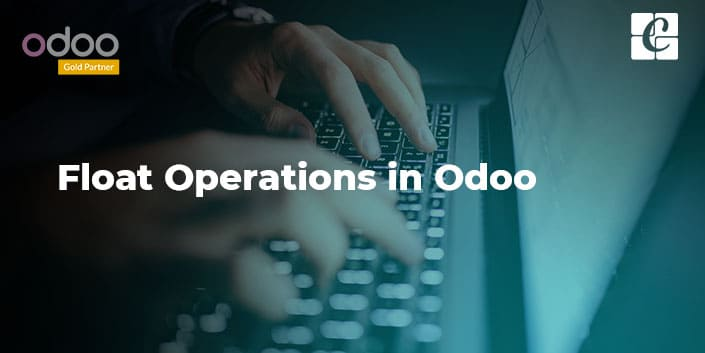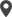Enable Dark Mode!By: Mashood K

# Float Operations in Odoo

## Technical

Odoo properly stores, retrieves, and manipulates records to ensure that the end-user gets the exact data they wish. Sometimes, float values(eg: 125.325) can have complexity to maintain its exact value in the system. This type of value can vary based on the environment and platforms. Odoo brings well-defined float management tools to maintain float reliability. Today we are going to take a look at those float tools and how they work.

## 1) Decimal Accuracy

Decimal accuracy is a measurement tool of Odoo to let the end-user specify the floating position of different categories like unit of measure, price. This feature helps the user to decide how many values to be displayed as decimals in each category. Let’s check its usage by using an example.Here you can see, we have set decimal accuracy for ‘Product Unit of Measure’ as 5. It means that wherever Odoo shows unit of measure, it will show 5 decimal points as you see below.Now, let’s check the coding part.

We have to import decimal_precision which is a module to specify decimal accuracies.

`from odoo.addons import decimal_precision as dp`

Or we can do it by referring to the corresponding module.

`precision = self.env['decimal.precision'].precision_get('Product Unit of Measure')`

Then we can use it anywhere like field declaration, functions, etc

`product_qty = fields.Float(string='Quantity', digits=dp.get_precision('Product Unit of Measure'), required=True)`

Each time when the system renders the view, Odoo searches for the corresponding decimal position and applies it to the related field.

Methods to check decimal points

Odoo provides some tools to check the decimal position of value. Here, we are checking those methods and functions used to check decimal point conditions.

float_compare()

Float_compare is a function that is defined in the odoo tools directory. This function is useful while we need to compare a value with another. In other words, This function determines value is lower than or greater than of another value by considering the given precision, precision rounding, etc.

Parameters

value1(float): First value to be checked

value2(float): Second value to be checked

preceision_digits(integer): Number of fractional digits to be checked .default value is None.

preceision_rounding(integer): After the precision value to be rounded .default value is None.

Return

-1 : If the first value is less than the second value.

0 : If the first value is equal to the second value.

1 : If the first value is greater than the second value.

`value1 = 5.125value2 = 7.548if float_compare(value1, value2, precision_rounding=self.product_uom.rounding) <= 0:print('value1 is less than value2')elif float_compare(value1, value2, precision_rounding=self.product_uom.rounding) == 0:print('value1 is equal to value2')else:print('value1 is greater than value2')`

float_is_zero()

This function is also defined in the odoo tools. It returns true if the given value can be considered as zero with the given precision value and rounding digits.

Parameters

value(float): Value to be checked whether it is zero or not.

preceision_digits(integer): Number of fractional digits to be checked .default value is None.

preceision_rounding(integer): After the precision value to be rounded .default value is None.

Return

True if the given value is zero. Else False.

float_round()

This is also defined in the Odoo tools directory which lies in the float_utils.py. This function returns the given value by rounding it with the specified rounding criteria.

Parameters

value(float): Value to be rounded.

preceision_digits(integer): Number of fractional digits to be rounded .default value is None.

preceision_rounding(integer): After the precision value to be rounded .default value is None.

Return

Returns the rounded values.

float_repr()

This function returns the string representation of the given value with the given decimal precision. This is used to get the string value of a float. Don’t call it for rounding. This may lead to getting the wrong value. Instead, you can use the float_round().

Parameters

value(float): Value to be converted to.

preceision_digits(integer): Number of fractional digits to be rounded.

Return

String representation of the given float value.

float_split_str()

This function will be useful when you need to split the integer value and fraction values from a float value. It exactly split the given value in it’s unitary and decimal parts. The float value is first rounded by using the function float_round() which we have discussed above. Then the rounded value converts to string by using the function float_repr(). Then it will split the value and returns a tuple which contains both integer and fractional parts.

Parameters

value: Value to be converted.

preceision_digits(integer): Number of fractional digits to be rounded.

Return

Converted values as strings in a tuple.

float_split()

This function behaves the same as the float_split_str() except it returns integer values instead of string values.

Parameters

value: Value to be converted.

preceision_digits(integer): Number of fractional digits to be rounded.

Return

Converted values as integers in a tuple.

If you need any assistance in odoo, we are online, please chat with us.

### Recent Posts

#### How to Configure Chatbots & Canned Responses in Odoo 16Calicut

Cybrosys Technologies Pvt. Ltd.
Neospace, Kinfra Techno Park
Kakkancherry, Calicut
Kerala, India - 673635London

Cybrosys Limited
Alpha House,
100 Borough High Street, London,
SE1 1LB, United KingdomKochi

Cybrosys Technologies Pvt. Ltd.
1st Floor, Thapasya Building,
Kochi, India - 682030.Bangalore

Cybrosys Techno Solutions
The Estate, 8th Floor,